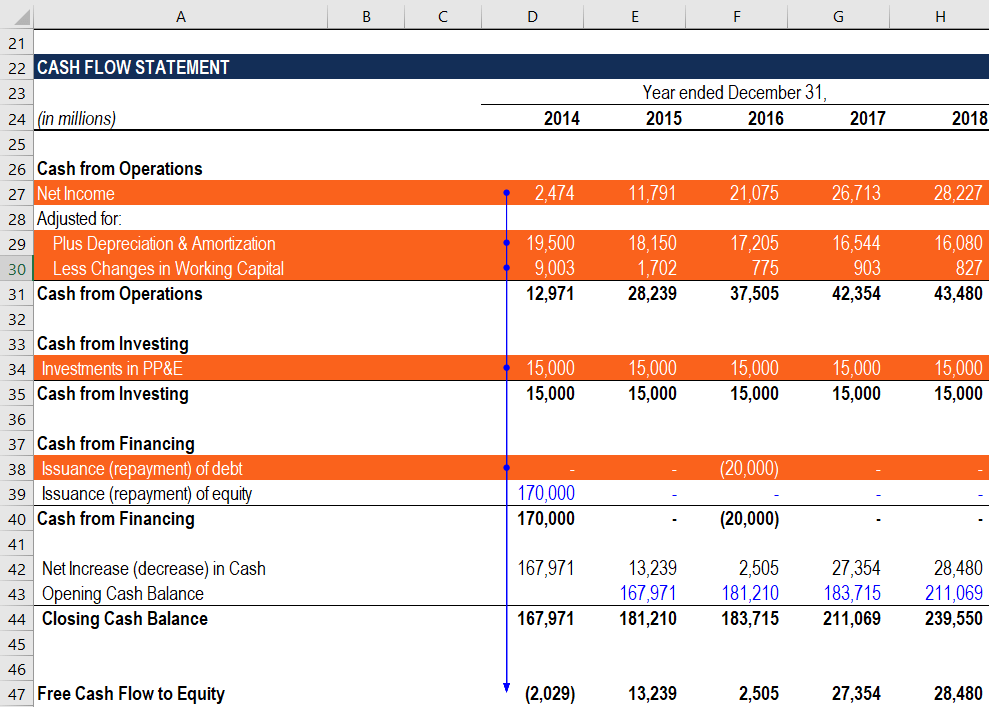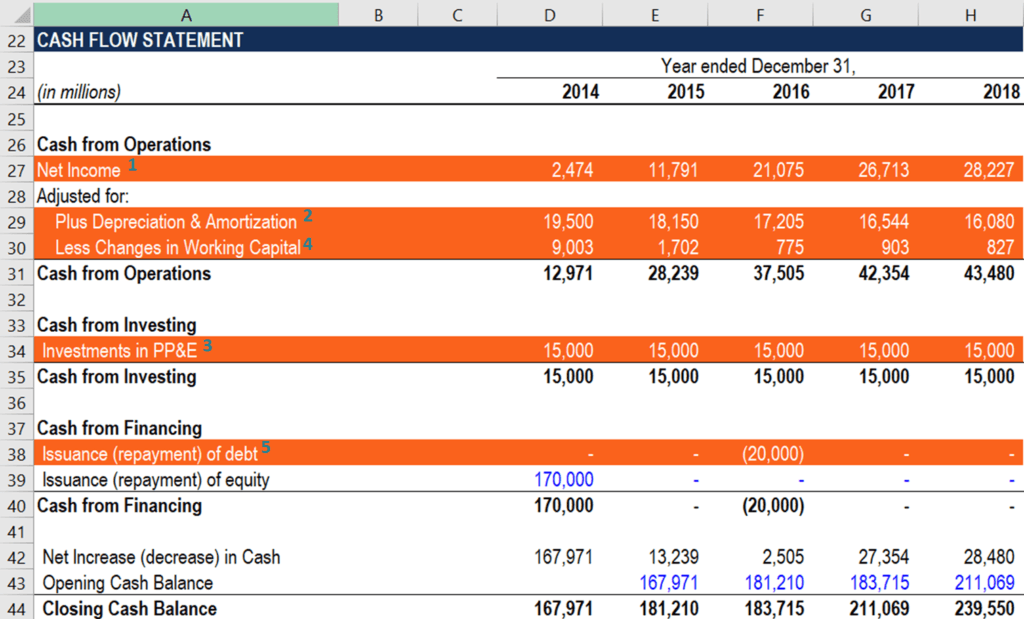# How to Calculate FCFE from Net Income

FCFE = Net Income + Depreciation & Amortization – CapEx – ΔWorking Capital + Net Borrowing

## How to Calculate FCFE from Net Income

Free Cash Flow to Equity (FCFE) can be calculated using net income as well as using the Free Cash Flow to the Firm (FCFF) formula. It is the amount of cash generated by a company that can be potentially distributed to the company’s shareholders. When using an intrinsic valuation method such as the Discounted Cash Flow (DCF) valuation model, an analyst can use FCFE as the business’ cash flow generating ability.

The FCFE is different from the free cash flow to the firm (FCFF), which indicates the amount of cash generated to all holders of the company’s securities (both investors and lenders).### FCFE from Net Income Formula

Free cash flow to equity (FCFE) can be calculated in many ways. To calculate the FCFE from net income, we need to look at the formula and break it down. Here is the formula to calculate FCFE from net income:

##### FCFE = Net Income + Depreciation & Amortization – CapEx – ΔWorking Capital + Net Borrowing

However, FCFE is usually derived by using the free cash flow to the firm (FCFF) formula. To reconcile this, let’s look at how we get FCFE from FCFF. Here is the formula for FCFF:

##### FCFF = Net Income + Depreciation & Amortization – CapEx – ΔWorking Capital + Interest Expense (1 – t)

Where:

• FCFF – Free Cash Flow to the Firm
• CapEx – Capital Expenditure
• ΔWorking Capital – Net change in the Working Capital
• t – Tax rate

Notice that FCFE and FCFF share very similar terms such as depreciation, capital expenditures, and changes in working capital. The main difference between the FCFF and FCFE is the impact of interest expenses and their tax shields. Therefore, the FCFE can be calculated using the FCFF formula:

### FCFE from Net Income Formula and Financial Statements

An analyst who calculates the free cash flows to equity in a financial model must be able to quickly navigate through a company’s financial statements. The primary reason is that all the inputs required for the calculation of the metric are taken from the financial statements. The guidance below will help you to quickly and correctly incorporate the FCFE from Net Income calculation into a financial model.

1. Net Income: Net income (also referred to as the net earnings) can be found at the bottom of the income statement. In addition, the net income is listed on the cash flow statement in the calculation of the cash flows from operating activities. Every calculation of the cash flow from operating activities starts with the net income. Since many other inputs are taken from the cash flow statement as well, it is recommended to use the financial statement to link the net income to the FCFE calculations.
2. Depreciation & Amortization: The depreciation and amortization expense is recorded on the company’s income statement under the Expenses section. The section follows the company’s gross profit. Similar to net income, the depreciation and amortization expense is also listed on the cash flow statement on the Cash from Operations section.
3. CapEx: The capital expenditure (CapEx) can be found on the cash flow statement within the Cash from Investing section.
4. Change in working capital (can also be denoted as ΔWorking Capital) is calculated in the company’s cash flow statement within the Cash from Operations section.
5. Net debt: The net debt amount is also located on the cash flow statement under the Cash from Investing section.### More Resources

We hope you’ve enjoyed CFI’s explanation of how to calculate FCFE from net income. CFI is the official provider of the Financial Modeling and Valuation Analyst (FMVA)™ certification program, designed to transform anyone into a world-class financial analyst.

To keep learning and developing your knowledge of financial analysis, we highly recommend the additional CFI resources below:

• Capital Expenditure
• How the 3 Financial Statements are Linked
• Projecting Income Statement Line items
• Owner’s Equity